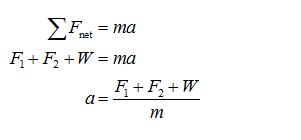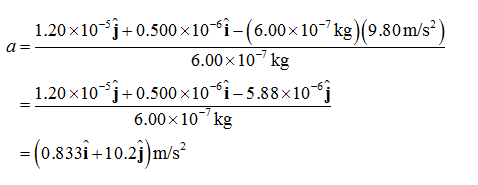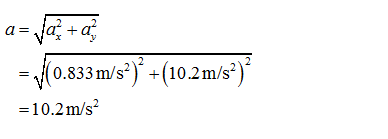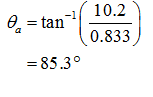# A flea jumps by exerting a force of 1.20×10−5N straight down on the ground. A breeze blowing on the flea parallel to the ground exerts a force of 0.500×10−6N on the flea. Find the direction and magnitude of the acceleration of the flea if its mass is 6.00×10−7kg. Do not neglect the gravitational force.

Question
8 views

A flea jumps by exerting a force of 1.20×10−5N straight down on the ground. A breeze blowing on the flea parallel to the ground exerts a force of 0.500×10−6N on the flea. Find the direction and magnitude of the acceleration of the flea if its mass is 6.00×10−7kg. Do not neglect the gravitational force.

check_circle

Step 1

According to the Newton’s second law, the net force acted on the flea is,Substitute the values, to find the acceleration of the flea.Step 2

The magnitude of the acceleration is,The direction of the acceleration is,...

### Want to see the full answer?

See Solution

#### Want to see this answer and more?

Solutions are written by subject experts who are available 24/7. Questions are typically answered within 1 hour.*

See Solution
*Response times may vary by subject and question.
Tagged in

### Physics